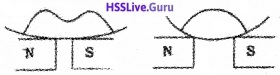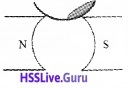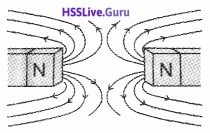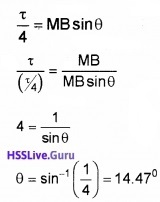# Plus Two Physics Chapter Wise Questions and Answers Chapter 5 Magnetism and Matter

Students can Download Chapter 5 Magnetism and Matter Questions and Answers, Plus Two Physics Chapter Wise Questions and Answers helps you to revise the complete Kerala State Syllabus and score more marks in your examinations.

## Kerala Plus Two Physics Chapter Wise Questions and Answers Chapter 5 Magnetism and Matter

### Plus Two Physics Magnetism and Matter NCERT Text Book Questions and Answers

Question 1.
Answer the following questions regarding earth’s magnetism:

1. A vector needs three quantities for its specification. Name the three independent quantities conventionally used to specify the earth’s magnetic field.
2. The angle of dip at a location in southern India is about 18°. Would you expect a greater or lesser dip angle in Britain?
3. If you make a map of magnetic field lines at Melbourne in Australia, would the lines seen to go into the ground or come out of the ground?
4. In which direction would a compass free to move in the vertical plane point to, if located right on the geomagnetic north or south pole?
5. The earth’s field is claimed roughly approximately, the field due to a dipole magnetic moment 8 × 1022 JT-1 located at the centre. Check your or¬der of magnitude of this number same way.
6. Geologists claim that besides the main magnetic NS poles, there are several local poles on the earth’s surface oriented in different directions. How is such a thing possible at all?

1. Magnetic declination, angle of dip, horizontal component of earth’s magnetic field.

2. Greater angle of dip in Britain, (it is about 70°), because Britain is closer to the magnetic north pole.

3. Field lines of B due to the earth’s magnetism would seem to come out of the ground.

4. A compass is free to move in a horizontal plane, while the earth’s field is exactly vertical at the magnetic poles. So the compass can point in any direction there.

5. Use the formula for field B on the normal bisector of a dipole of magnetic moment m.
B = $$\frac{\mu_{0}}{4 \pi} \frac{M}{r^{3}}$$
Take M = 8 × 1022 JT-1
r = 6.4 × 106 m;
one gets B = 0.3G
which checks with the orde of magnitude of the observed field on the earth.

6. The earth’s field is only approximately a dipole field. Local N-S poles may arise due to, for instance, magnetized minerals deposits.Question 2.
A short bar magnet of magnetic moment m = 0.32JT-1 is placed in a uniform external magnetic field of 0.15T. If the bar is free to rotate in the plane of the field, which orientations would correspond to its

• stable and
• unstable equilibrium? What is the potential energy of the magnet in each case?

Given M=0.32JT-1 B = 0.15T, U = ?

• If $$\overrightarrow{\mathrm{M}} \| \overrightarrow{\mathrm{B}}$$, then we have stable equilibrium and U = -MB = -0.32 × 0.15T = -4.8 × 10-2 J
• If $$\overrightarrow{\mathrm{M}}$$ is anti-parallel to $$\overrightarrow{\mathrm{B}}$$ , we have unstable equilibrium and
U = MB = 0.32 × 0.15J = 4.8 × 10-2J

Question 3.
A closely wound solenoid of 800 turns and area of cross-section 2.5 × 10-4 m2 carries a current of 3.0A. Explain the sense in which the solenoid acts like a bar magnet. What is its associated magnetic moment?
Given N = 800, A = 2.5 × 10-4 m2, I = 3.0A, M = ?
Since M = NIA
∴ M = 800 × 3 × 2.5 × 10-4
or M = 0.60JT-1 along the axis of the solenoid.Question 4.
A closely wound solenoid of 2000 turns and area of cross-section 1.6 × 10-4 m2, carrying a current of 4.0A, is suspended through its centre allowing it to turn in a horizontal plane.

1. What is the magnetic moment associated with the solenoid?
2. What is the force and torque on the solenoid if a uniform horizontal magnetic field of 7.5 × 10-2 is set up at an angle of 30° with the axis of the sole¬noid?

1. Magnetic dipole moment,
M = nIA
= 2000 × 4.0 × 1.6 × 10-4 = 1.28JT-1

2. Net force = 0
Torque, τ = MB sinθ
= 1.28 × 7.5 × 10-2 × sin 30°
= 1.28 × 7.5 × 10-3 × 1/2 = 4.8 × 10-2 Nm.Question 5.

1. Why does a paramagnetic sample display greater magnetization (for the same magnetizing field) when cooled?
2. Why is diamagnetism, in contrast, almost independent of temperature?
3. If a toroid used bismuth for its core, will the field in the core be (slightly) greater or (slightly) less than when the core is empty?
4.  If the permeability of a ferromagnetic material independent of the magnetic field? If not is it more for lower or higher fields?
5. Magnetic field lines are always nearly normal to the surface of a ferromagnet at every point.
6. Would the maximum possible magnetisation of a paramagnetic sample be of the same order of magnitude as the magnetisation of a ferromagnet?

1. The tendency to disrupt the alignment of dipoles (with the magnetising field) arising from random thermal motion is reduced at lower temperatures.

2. The induced dipole moment in a diamagnetic sample is always opposite to the magnetising field, no matter what the internal motion of the atom is.

3. Slightly less, since bismuth is diamagnetic.

4. No, as is evident from the magnetisation curve. From the slope of the magnetisation curve, it is clear that μ is greater for lower fields.

5. Proof of the important fact (of much practical use) is based on boundary conditions of magnetic fields (B & H) at the interface of two media. (When one of the media has μ>>1, the field lines meet this medium nearly normally).

6. Yes. Apart from minor differences in the strength of the individual atomic dipoles of two different materials, a paramagnetic sample with saturated magnetisation will have the same order of magnetisation. But of course, saturation requires impractically high magnetising fields.

### Plus Two Physics Magnetism and Matter One Mark Questions and Answers

Question 1.
Nickel shows ferromagnetic property at room temperature. If the temperature is increased beyond Curie temperature, then it will show
(a) anti ferromagnetism
(b) no magnetic property
(c) diamagnetism
(d) paramagnetism
(d) paramagnetismQuestion 2.
According to Curie’s law, the magnetic susceptibility of a substance at an absolute temperature T is proportional to
(a) 1/T
(b) T
(c) 1/T2
(d) T2
(a) 1/T
Explanation : According to Curie’s law X ∝ $$\frac{1}{T}$$Question 3.
Above Curie temperature
(a) a paramagnetic substance becomes ferromagnetic substance
(b) a ferromagnetic substance becomes paramagnetic
(c) a paramagnetic substance becomes diamagnetic
(d) a diamagnetic substance becomes paramagnetic
(b) a ferromagnetic substance becomes paramagnetic

### Plus Two Physics Magnetism and Matter Two Mark Questions and Answers

Question 1.
Classify the following properties into diamagnetic, paramagnetic and ferromagnetic.

1. Susceptibility -1≤x<0
2. In uniform magnetic field it acquires a large magnetisation in the direction of the field.
3. When it is suspended in a magnetic field, it will come to rest perpendiculartothe magnetic field.
4. Susceptibility of the substance varies inversely as temperature of the substance upto curie temperature ie. xm∝ $$\frac{1}{T}$$

• Dia – a,c
• Para – d
• Ferro – bQuestion 2.
Classify the following properties into diamagnetism and ferro magnetism.

1. In non uniform magnetic field, it more from high to law field.
2. Magnetic field lines are repelled from this material, If we place in a external magnetic field.
3. susceptibility greater than one, <0, +ve
4. Iron, Nickel, Cobalt are the examples

1. Diamagnetism
2. Diamagnetism
3. Ferro magnetism
4. Ferro magnetismQuestion 3.
Fill in the blanks.1. negative
2. Weak magnetic field to strong magnetic field.
3. Individual atoms have tiny magnetic moments
4. µr >1

### Plus Two Physics Magnetism and Matter Three Mark Questions and Answers

Question 1.
The figure shows hysteresis curves for soft iron and stell.1. Which among the two is the hysteresis loop of soft iron?
2. Which one among the two materials is preferred for use in transformers and galvanometers?
3. When steel is once magnetized, the magnetiza-tion is not easily destroyed even if it is exposed to strong reverse fields. Give reason.

1. Fig. b
2. Soft iron
3. When steel is magnetised the magnetic domains in the material is permanently set and magnetised permanently in the direction of the applied magnetic field.Question 2.
The figure shows a liquid placed on the pole pieces of two magnets.1. Which magnetic behaviour is exhibited by the liquid? (1)
2. Write any two characteristics of this magnetic behaviour? (1)
3. Does this behaviour transform with temperature. Why? (1)

1. Diamagnetism
2. characteristics of this magnetic behaviour:

• Permeability of a diamagnetic material is less than one.
• Susceptibility is small and negative.

3. No. The magnetic dipole moment induced in the diamagnetic material is opposite to the magnetising field and hence does not affect the thermal motion of atoms. Hence change in temperature has no effect on diamagnetism.

Question 3.
A magnetic material contained in a curved glass plate, when placed in a nonuniform magnetic field, exhibits a property as shown in figure.1. Which type of magnetic material is this? Explain the property
2. Write two examples for such a magnetic material. Explain how the property relates with temperature?
3. “The susceptibility of a material is small” what do you mean by this statement.

1. Diamagnetism: Diamagnetic materials are repelled from external magnetic field.
2. Bismuth, Copper, Lead, Nitrogen, Water. Diamagnetism is independent of temperature.
3. Diamagnetism developed by external magnetic field is very small.Question 4.
A place where dip = 90°, BH = 0

1. This place is at…………
2. What is the value of Bu at this place?
3. Can a magnetic needle align in the N-S direction at this place?

1. Magnetic pole.

2. B = $$\sqrt{B_{v}^{2}+B_{h}^{2}}$$
But Bh = 0
∴ B = Bv

3. No. Magnetic needle align verticallyQuestion 5.
Classify into diamagnet, Paramagnet and Fero magnet.

1. Feebly magnetized in the same direction
2. mr slightly more than none
3. does not allow lines of force
4. temperature independent
5. exhibit hysteresis
6. strongly attracted by a bar magnet

1. Paramagnet
2. Para magnet
3. Dia magnet
4. Diamagnet
5. Ferromagnet
6. Ferromagnet

### Plus Two Physics Magnetism and Matter Four Mark Questions and Answers

Question 1.
Magnetic field lines are the visualization of magnetic field.

1. Write any three properties of magnetic field lines.
2. The arrangement shows two bar magnets placed near each other. Draw the magnetic field lines of the system.1. properties of magnetic field lines:

• The magnetic field lines of a magnet form continuous closed loops.
• The tangent to the field line at a given point represents the direction of magnetic field at that point.
• Flux density of magnetic field represents the strength of magnetic field.
• The magnetic field lines do not intersect

2.Question 2.
The figure shows the magnetic field of earth.1. Identify the labels A, B, C. (1)
2. The lines drawn on a map through places that have the same declination are called………(1)
3. The horizontal component of earth’s magnetic field at a place is 0.25 × 10-4T and the resultant magnetic field is 0.5 × 10-4 T. Find the dip and the vertical component of the earth’s magnetic field at the place. (2)

1. the labels A, B, C:

• A – Magnetic equator
• B – Magnetic axis
• C – Declination

2. Isogonic lines3. Horizontal component of earth’s magnetic field is
BH = B cosδ
0.25 × 10-4 = 0.5 × 10-4 δ
δ = 60°
Vertical component of earth’s magnetic field is
Bv = B sin δ = 0.5 × 10-4 sin 60 = 0.43 × 10-4T.

### Plus Two Physics Magnetism and Matter Five Mark Questions and Answers

Question 1.
When a magnetic needle is placed in a non-uniform magnetic field it experiences
1.

• a force but no torque
• a torque but no force
• force and torque
• neither a force nor a torque (1)

2. A bar magnet held perpendicular to a uniform magnetic field as in the figure. If the torque acting on it is to be reduced to 1/4th by rotating the magnet towards the direction of the field, find the angle through which the magnet is to be rotated. (2)3. State whether the potential energy of the magnet increases or decreases after rotation. Justify your answer. (2)